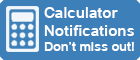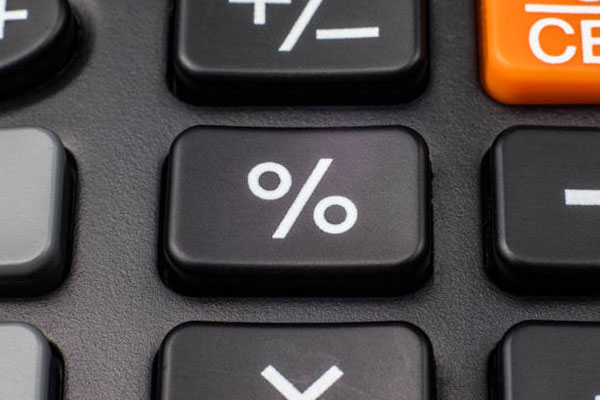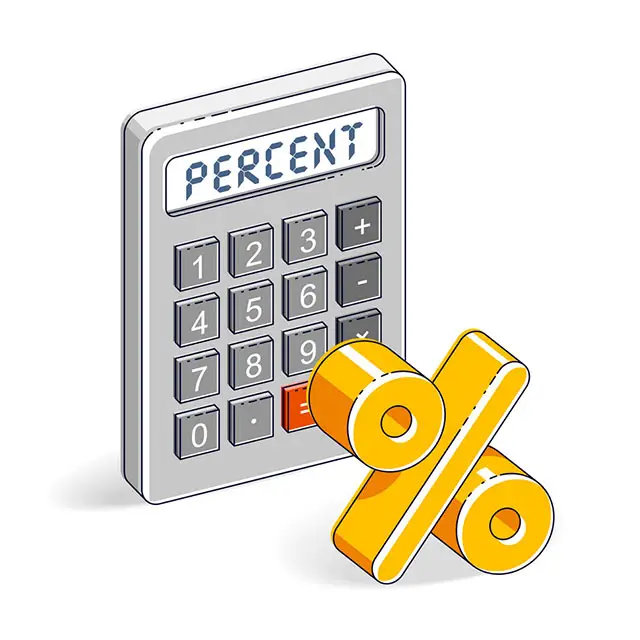Calculator Library
Free Online CalculatorsHome » 87 Calculators » Blog » Percentage Calculators

Huge Domain Name Sale | Free Domains
Domain names on sale at crazy prices. Register a new domain for FREE when you buy another service.

## PERCENTAGE CALCULATORSA percentage calculator is a tool used to perform mathematical operations involving percentages, such as calculating a percentage of a given number, calculating what percentage one number is of another or calculating the percentage variation from one number to another. This type of calculator is commonly used in many settings, such as in finance, business and education.

In this article, we'll explore some of the key features and capabilities of percentage calculators and how they can be used to perform various percentage calculations.

## What Are Percentages?

Percentages are ratios that represent fractions of 100, so the word "percent" really means "per 100". For example, if you have 10 apples and you give away 4 of them, you can say that you've given away 40% of your apples. A value written as a percentage can also be written as a decimal or a fraction. For example, we know that a quarter is 25%, but this can also be expressed as 0.25 or 25100, which reduces to 14.

## Calculating Percentages

The basic formula to calculate percentages is % = (Value ÷ Total Value) × 100.

Simple percentage calculations are easy to do in your head. For example, 40% of 100 is obviously 40; 25% (a quarter) of 20 is 5. There are also a few tricks you can use to make percentage calculations easier:

1. One of the easiest ways to calculate percentages uses the Swap Method. For example, 28% of 50 and 50% of 28 both give the same result of 14, but the second option is much easier to calculate. This method works for all percentages.
2. Another easy percentage calculation uses the 1% Method. First, divide the total value by 100 to calculate 1%. This simply moves the decimal point two places to the left. For example, 1% of 400 is 400 ÷ 100 = 4. Then, you can calculate any percentage you need by multiplying that value to get your final result. Continuing this example, 25% of 400 is 4 x 25 = 100.

However, using a percentage calculator is usually much quicker and easier.

## Using Percentage Calculators

A percentage calculator can save you time and effort when performing these calculations. For example, you can find our free online Percentage Calculator here. Many calculators have a dedicated percentage button that allows you to quickly and easily perform percentage calculations. Other calculators may require you to enter the numbers and perform the calculation using the appropriate mathematical operations.

To use a percentage calculator, you first need to enter the number that you want to find the percentage of. This is called the "base" number. Next, you need to enter the percentage that you want to calculate. This is called the "percentage" number. Finally, press the "calculate" button to see the result. For example, if you want to determine what 40% of 100 is, you would enter "100" as the base number and "40" as the percentage number. The calculator would then show you that 40% of 100 is 40.

### Finance Percentages

One of the most common uses for a percentage calculator is in finance. For example, if you're trying to calculate the interest on a loan, you can use a percentage calculator to determine the amount of interest you'll be charged. You can also use a percentage calculator to determine the amount of sales tax you'll be required to pay on a purchase.

In business, percentage calculators are commonly used for a variety of purposes. For example, suppose you're trying to determine the profit margin on a product. In that case, you can use a percentage calculator to calculate markups on prices or the percentage of the price that represents the profit. You can also use a percentage calculator to determine the percentage of a company's revenue generated by a particular product or service.

### Education Percentages

In education, students often use percentage calculators to help them understand and solve math problems and check their work. Percentage calculators can also be used to calculate grades or test scores. For example, a student may use a percentage calculator to determine their class grade based on the number of marks they've earned.

### Everyday Percentages

In daily life, individuals often use percentage calculators to perform everyday calculations, such as working out the sale price of an item or deciding how much tip to leave on a restaurant bill. A tip calculator is simply a type of percentage calculator.One of the advantages of using a percentage calculator is that it can save you time and effort. Rather than doing the calculations manually, you can simply enter the numbers into the calculator and let it do the work for you. This can be especially useful when dealing with larger numbers or complex calculations as it allows you to quickly find the percentage of a given number without having to perform the calculation by hand.

Another advantage of using a percentage calculator is that it can help you avoid making mistakes. When doing calculations by hand, it's easy to make mistakes, such as accidentally transposing numbers or forgetting to carry a digit. With a percentage calculator, you can be confident that your calculations are accurate.

Additionally, because percentage calculators are often designed with user-friendly interfaces and easy-to-use controls, they can be used by people of all ages and skill levels.

## Types Of Percentage Calculators

There are many different types of percentage calculators available. Some are simple and only allow you to calculate a single percentage, while others are more advanced and have additional features that make working with percentages even easier. For example, some calculators allow you to round the result of a calculation to a specific number of decimal places, which can be helpful when working with large numbers.

Other calculators may also include additional functions or enable more complex mathematical operations, such as the ability to convert percentages to decimals or fractions, convert between different measurement units or calculate the percentage of a number that falls within a given range. Some percentage calculators are available as more advanced software programs, such as desktop software or smartphone apps, while others can be found online.

### Online Percentage Calculators

Online percentage calculators are virtual tools accessed through the internet and can be used on any device with an internet connection. These calculators are often more versatile and powerful than their physical counterparts and may offer a broader range of functions and features. Some online percentage calculators even allow users to save and access their calculations later, making them a convenient option for individuals who need to perform multiple calculations involving percentages.

There are some limitations to using a percentage calculator. For example, some calculators may not be able to handle huge numbers or complex calculations. In these cases, doing the calculations manually or using a more advanced calculator may be necessary.

It's also necessary to be aware of rounding errors when using percentages. For example, numbers 1-4 are usually rounded down, while numbers 5-9 are rounded up. This can lead to mistakes:

 First, take a list of exact percentages... ...then round to the nearest whole number. 29.563% 30% 27.705% 28% 42.732% 43% TOTAL: 100.000% TOTAL: 101%

In this example, rounding produces an impossible result of 101%.

Overall, a percentage calculator is useful for anyone who needs to do calculations involving percentages. Whether you're a student, a businessperson or just someone who wants to quickly and easily calculate percentages, a percentage calculator can be a valuable tool to have on hand.

 Previous Post« The Google Calculator Next PostHistory Of The Calculator »